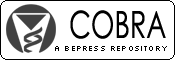## COBRA Preprint Series

#### Title

Mean Survival Time from Right Censored Data

#### Abstract

A nonparametric estimate of the mean survival time can be obtained as the area under the Kaplan-Meier estimate of the survival curve. A common modification is to change the largest observation to a death time if it is censored. We conducted a simulation study to assess the behavior of this estimator of the mean survival time in the presence of right censoring.

We simulated data from seven distributions: exponential, normal, uniform, lognormal, gamma, log-logistic, and Weibull. This allowed us to compare the results of the estimates to the known true values and to quantify the bias and the variance. Our simulations cover proportions of random censoring from 0% to 90%.

The bias of the modified Kaplan-Meier mean estimator increases with the proportion of censoring. The rate of increase varied substantially from distribution to distribution. Distributions with long right tails (log-logistic, log normal, exponential) increased the quickest (i.e., at lower censoring proportions). The other distributions are relatively unbiased until around 60% censoring. The Normal distribution remains unbiased up to 90% censoring.

Thus, the behavior of the modified Kaplan-Meier mean estimator depends heavily on the nature of the distribution being estimated. Since we rarely have knowledge of the underlying true distribution, care must be taken when estimating the mean from censored data. With modest censoring, estimates are relatively unbiased, but as censoring increases so does the bias. With 30% or more censoring the bias may be too high. This is in contrast to the Kaplan-Meier estimator of the median which is relatively unbiased.

#### Disciplines

Survival Analysis

COinS